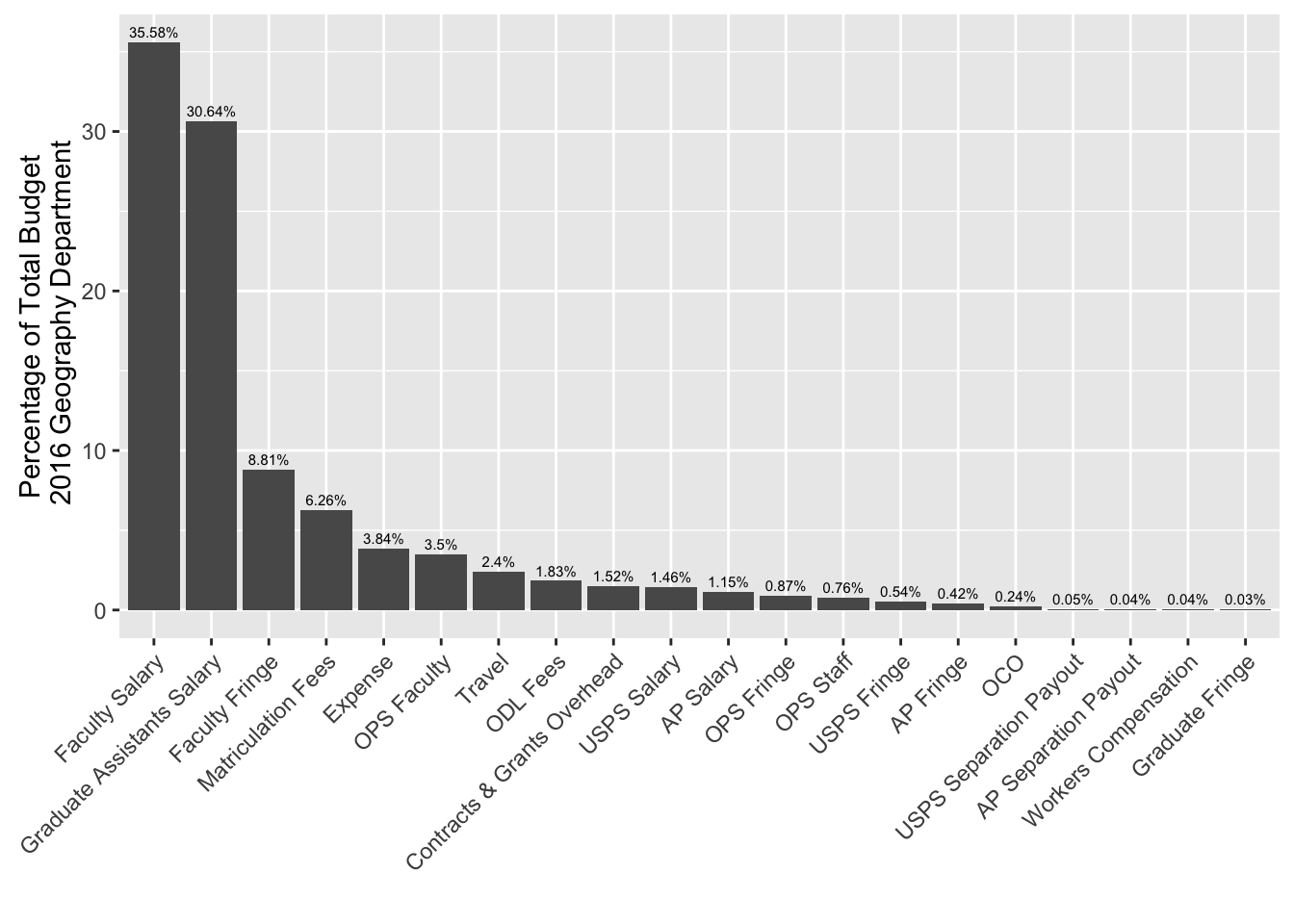# Geography Budget 2016

Let’s look back on how the department spent its money during my first year as chair. The data for Fiscal Year 2016 were tabulated by Audrey Nichols our Office Manager and Financial Assistant. She asked me to illustrate the categories using a graph. I decided comparisons are easiest to make using an ordered bar graph.

The largest expenditures are faculty and graduate student salaries accounting for nearly two thirds of our budget. Note that the categories of AP, USPS, and OPS are for our staff (Audrey, Alex, and Krystal). I think the university needs to pay its quality staff much better. This is a point of emphasis for me this year.

Here is the R code I used to make the graph.

Data

``````Cat = c(
"Faculty Salary",
"Faculty Fringe",
"AP Salary",
"AP Separation Payout",
"AP Fringe",
"USPS Salary",
"USPS Separation Payout",
"USPS Fringe",
"OPS Faculty",
"OPS Staff",
"OPS Fringe",
"Travel",
"Expense",
"Workers Compensation",
"Matriculation Fees",
"OCO",
"ODL Fees"
)

Perc = c(
35.58,
8.81,
1.15,
0.04,
0.42,
1.46,
0.05,
0.54,
30.64,
3.5,
.76,
.87,
.03,
2.4,
3.84,
.04,
6.26,
.24,
1.52,
1.83
)

df = data.frame(Category = factor(Cat),
Percentage = Perc)``````

Plot

``````library("ggplot2")

ggplot(df, aes(x = reorder(Category, -Percentage), y = Percentage)) +
geom_bar(stat = "identity") +
geom_text(aes(label = paste(Percentage, "%", sep = "")), vjust = -.5, size = 2) +
xlab("") + ylab("Percentage of Total Budget\n 2016 Geography Department") +
theme(axis.text.x = element_text(angle = 45, hjust = 1))``````